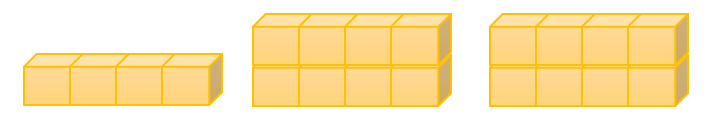Mathematics
Easy

Question

# Jim creates a pattern using the rule “Each layer has 4-blocks”.The number of cubes in the 10th shape in Lin’s pattern is

## 40    60    80    100Hint:

## The correct answer is: 40

### We are given a pattern with number of cubes. The rule used to make the pattern is “Each layer has four blocks”.One layer is added every time. With each layer, 4 blocks are added.The number of cubes in the first shape is 4.The number of cubes in the second shape is (2 × 4) = 8The number of cubes in the third shape is (3 × 4) = 12So, the number of cubes in the 10th shape will be 10 × 4 = 40.

We have to be careful about the order of the sequence.

### Related Questions to study#### With Turito Foundation.#### Get an Expert Advice From Turito.